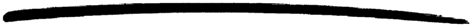# math

math

### MODULE SUMMARY

Mathematical Functions

### DESCRIPTION

This module provides an interface to a number of mathematical functions.

Note

Not all functions are implemented on all platforms. In particular, the erf/1 and erfc/1 functions are not implemented on Windows.

### EXPORTS

A useful number.

Types:

X = Y = number()

A collection of math functions which return floats. Arguments are numbers.

Types:

X = number()

Returns the error function of X, where

```erf(X) = 2/sqrt(pi)*integral from 0 to X of exp(-t*t) dt.
```

Types:

X = number()

erfc(X) returns 1.0 - erf(X), computed by methods that avoid cancellation for large X.

### Bugs

As these are the C library, the bugs are the same.

stdlib 1.16.3